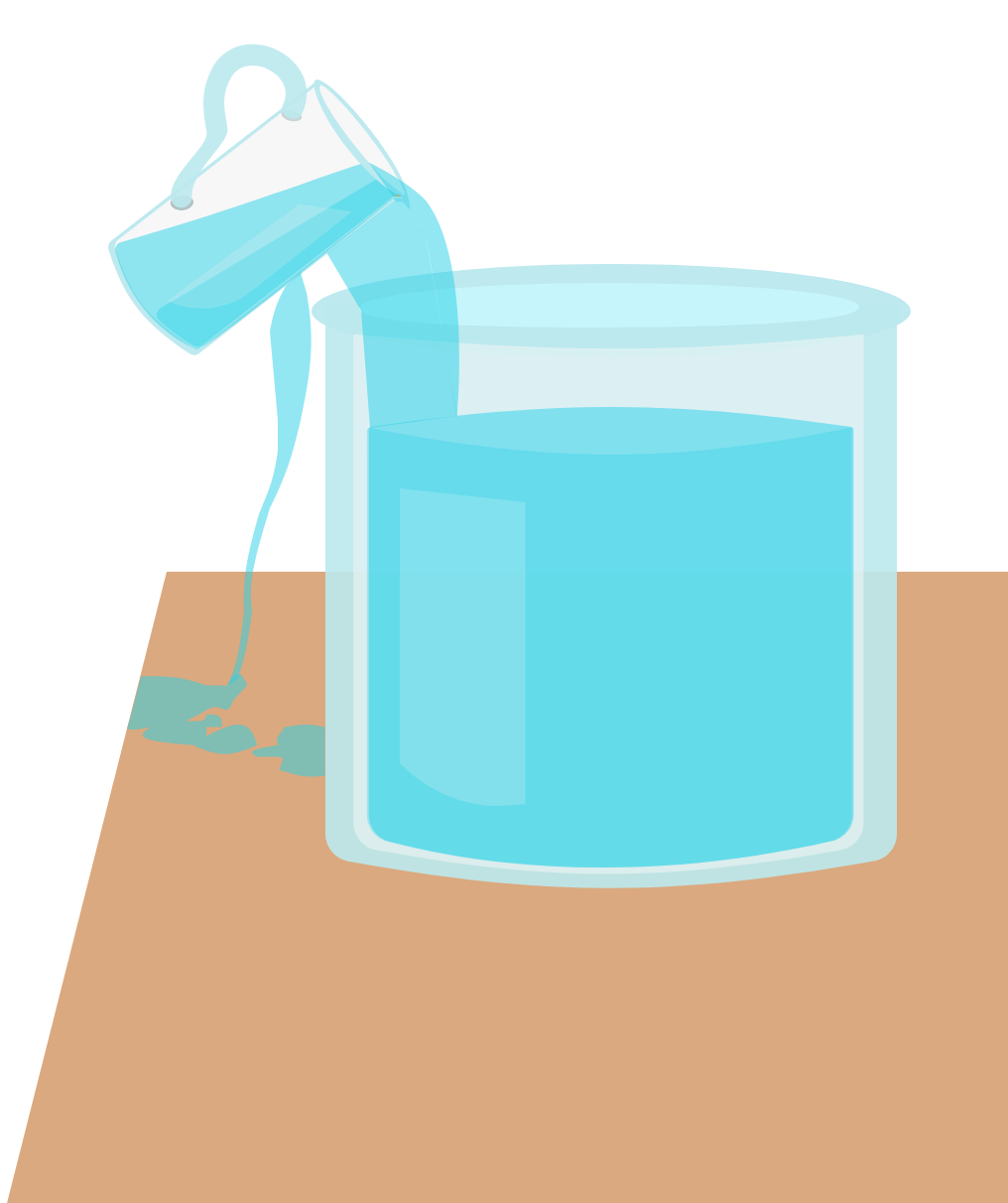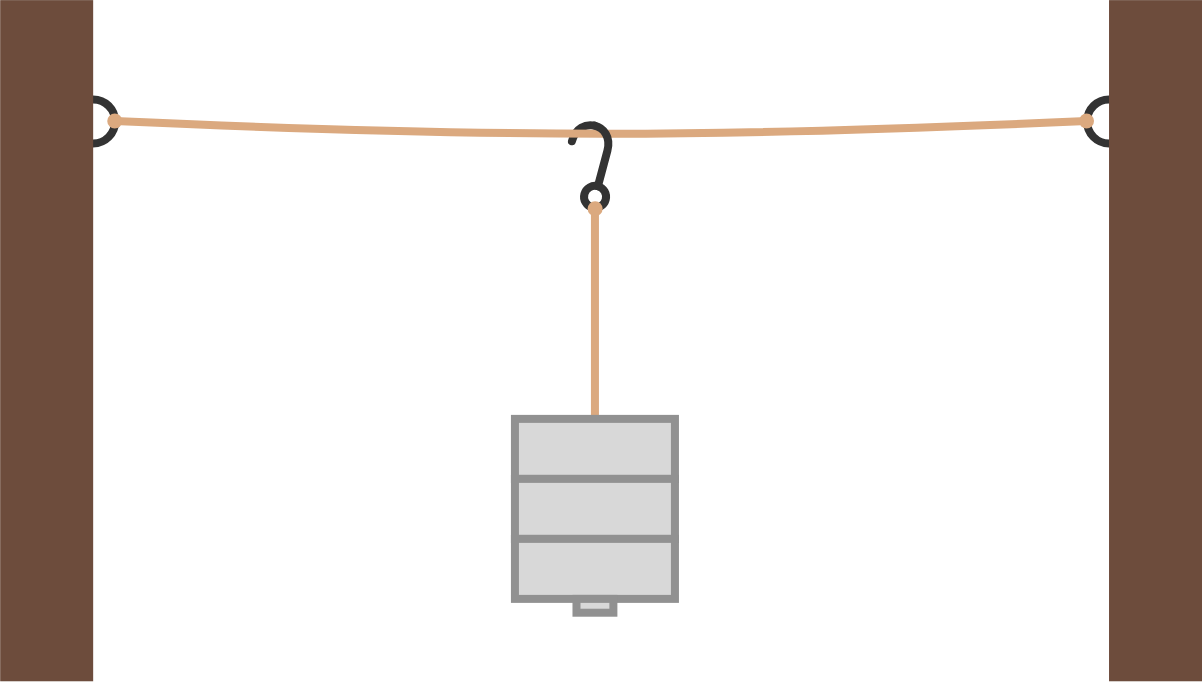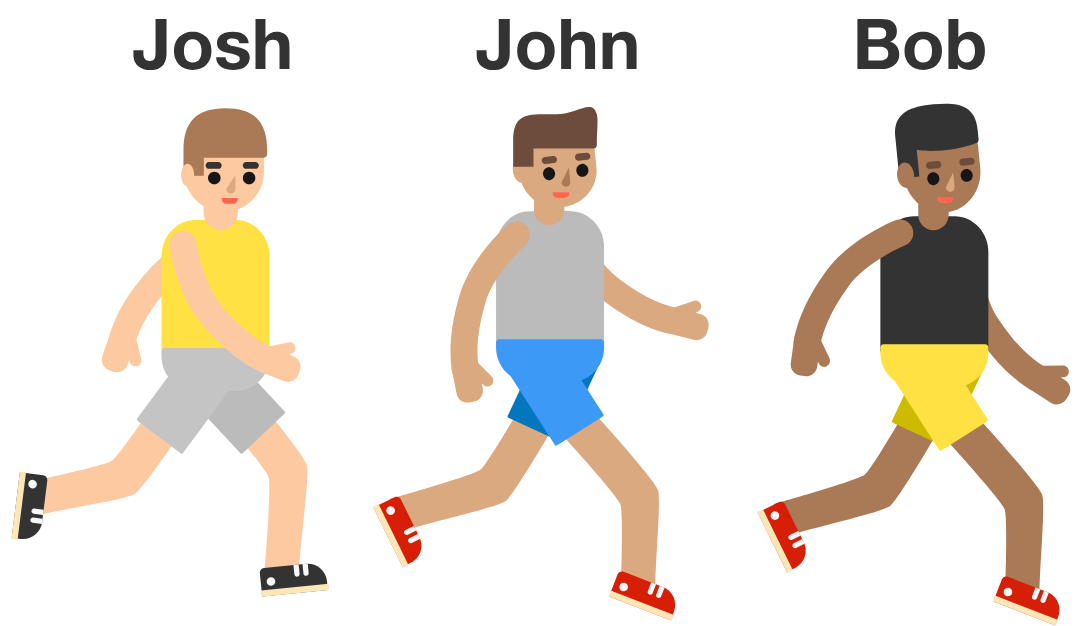# Problems of the Week

Contribute a problem

# 2018-11-26 Basic

When liquid is poured from a container (e.g. tea poured from one teacup to another), the liquid often slides along the side of the container, making a real mess.

What can be done to prevent this?The numbers in the rectangles represent their respective areas.

What is the area of the fourth rectangle?

Charlie hangs a string horizontally between two walls and uses an identical string to hang weights vertically.

As he adds weights, the tensions in both strings increase.

Which string will break first?Assume that the string is made from ordinary twine, which stretches minimally under tension.

Bob, John, and Josh are racing in a $1 \si{\kilo\meter}$ competition. Each competitor runs at a constant rate.

• After Bob has run $\frac14 \si{\kilo\meter},$ Bob is ahead of John by $25 \si{\meter}.$
• After John has run $\frac12 \si{\kilo\meter},$ John is ahead of Josh by $20 \si{\meter}.$

When Bob finishes the race, how much does he beat Josh by?An involution is a function, $f(x),$ that "un-does" itself such that $f\big(f(x)\big) = x.$

Here are three examples:

$\begin{array}{lcl} f(x) = \frac{1}{x} & \implies & f\big(f(x)\big) = \frac{1}{\hspace{2mm} \frac{1}{x}\hspace{2mm} } = x \\ \\ f(x) = -x & \implies & f\big(f(x)\big) = -(-x) = x \\ \\ f(x) = \frac{x}{x-1} & \implies & f\big(f(x)\big) = \frac{\frac{x}{x-1}}{\frac{x}{x-1}-1} = x. \end{array}$

How many of these special types of functions are there?

×